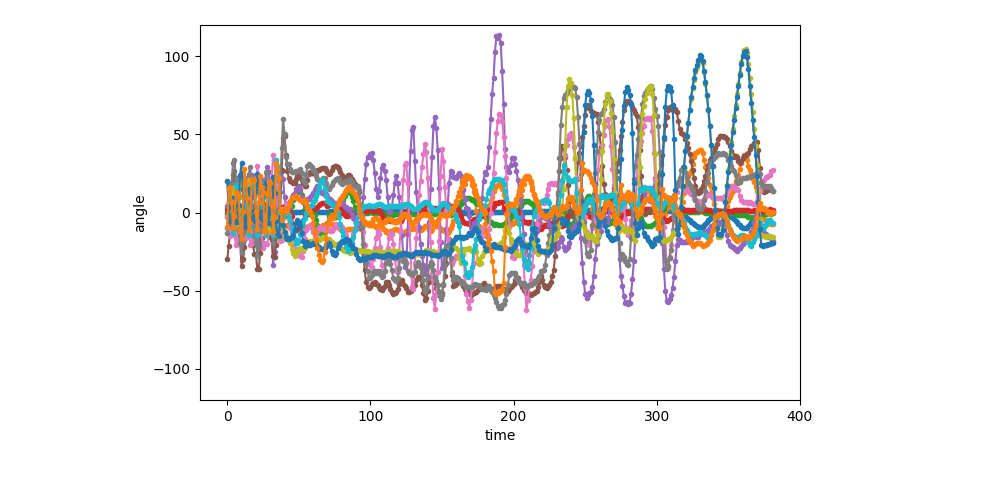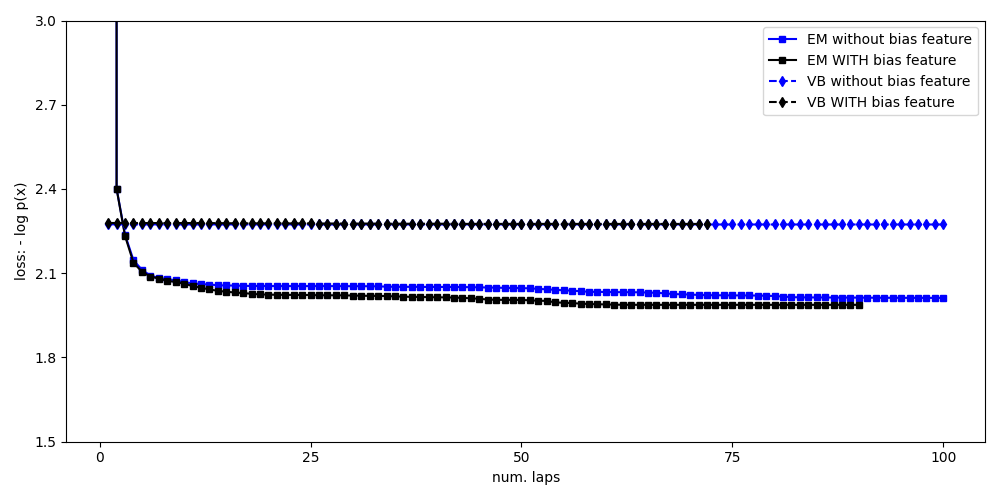# Visualizing learned state sequences and transition probabilities¶

Train a sticky HMM model with auto-regressive Gaussian likelihoods on small motion capture data. Discover how the likelihood hyperparameters might impact performance.

# sphinx_gallery_thumbnail_number = 3

import bnpy
import numpy as np
import os

import matplotlib
from matplotlib import pylab
import seaborn as sns

np.set_printoptions(suppress=1, precision=3)

FIG_SIZE = (10, 5)
pylab.rcParams['figure.figsize'] = FIG_SIZE


Load dataset from file

dataset_path = os.path.join(bnpy.DATASET_PATH, 'mocap6', 'dataset.npz')

dataset = bnpy.data.GroupXData.read_npz(dataset_path)
dataset_biasfeature = bnpy.data.GroupXData(
X=dataset.X,
Xprev=np.hstack([dataset.Xprev, np.ones((dataset.X.shape, 1))]),
doc_range=dataset.doc_range)


## Setup: Function to make a simple plot of the raw data¶

def show_single_sequence(
seq_id,
zhat_T=None,
z_img_cmap=None,
ylim=[-120, 120],
K=5,
left=0.2, bottom=0.2, right=0.8, top=0.95):
if z_img_cmap is None:
z_img_cmap = matplotlib.cm.get_cmap('Set1', K)

if zhat_T is None:
nrows = 1
else:
nrows = 2
fig_h, ax_handles = pylab.subplots(
nrows=nrows, ncols=1, sharex=True, sharey=False)
ax_handles = np.atleast_1d(ax_handles).flatten().tolist()

start = dataset.doc_range[seq_id]
stop = dataset.doc_range[seq_id + 1]
# Extract current sequence
# as a 2D array : T x D (n_timesteps x n_dims)
curX_TD = dataset.X[start:stop]
for dim in xrange(12):
ax_handles.plot(curX_TD[:, dim], '.-')
ax_handles.set_ylabel('angle')
ax_handles.set_ylim(ylim)
z_img_height = int(np.ceil(ylim - ylim))
pylab.subplots_adjust(
wspace=0.1,
hspace=0.1,
left=left, right=right,
bottom=bottom, top=top)
if zhat_T is not None:
img_TD = np.tile(zhat_T, (z_img_height, 1))
ax_handles.imshow(
img_TD,
interpolation='nearest',
vmin=-0.5, vmax=(K-1)+0.5,
cmap=z_img_cmap)
ax_handles.set_ylim(0, z_img_height)
ax_handles.set_yticks([])

bbox = ax_handles.get_position()
width = (1.0 - bbox.x1) / 3
height = bbox.y1 - bbox.y0
cax = fig_h.add_axes([right + 0.01, bottom, width, height])
cbax_h = fig_h.colorbar(
ax_handles.images, cax=cax, orientation='vertical')
cbax_h.set_ticks(np.arange(K))
cbax_h.set_ticklabels(np.arange(K))
cbax_h.ax.tick_params(labelsize=9)

ax_handles[-1].set_xlabel('time')
return ax_handles


## Visualization of the first sequence (1 of 6)¶

show_single_sequence(0)## Setup training: hyperparameters¶

K = 5            # Number of clusters/states

# Allocation model (Standard Bayesian HMM with sticky self-transitions)
transAlpha =   0.5  # trans-level Dirichlet concentration parameter
startAlpha =  10.0  # starting-state Dirichlet concentration parameter
hmmKappa   =  50.0  # set sticky self-transition weight

# Observation model (1st-order Auto-regressive Gaussian)
sF = 1.0          # Set observation model prior so E[covariance] = identity
ECovMat = 'eye'

nTask = 1


## Train with EM an HMM with AutoRegGauss observation model¶

Train single model using likelihood without any free parameter

$x_t ~ mbox{Normal}( A_k x_t-1, Sigma_k)$

Take the best of 10 random initializations (in terms of evidence lower bound).

bias0_trained_model, bias0_info_dict = bnpy.run(
dataset,
'FiniteHMM', 'AutoRegGauss', 'EM',
output_path=(
'/tmp/mocap6/bias0-K=%d-model=HMM+AutoRegGauss-ECovMat=1*eye/'
% (K)),
nLap=100, nTask=nTask, convergeThr=0.0001,
transAlpha=transAlpha, startAlpha=startAlpha, hmmKappa=hmmKappa,
sF=sF, ECovMat=ECovMat, MMat='eye',
K=K, initname='randexamples',
printEvery=25,
)


Out:

Dataset Summary:
GroupXData
size: 6 units (documents)
dimension: 12
Allocation Model:  None
Obs. Data  Model:  Auto-Regressive Gaussian with full covariance.
Obs. Data  Prior:  MatrixNormal-Wishart on each mean/prec matrix pair: A, Lam
E[ A ] =
[[1. 0.]
[0. 1.]] ...
E[ Sigma ] =
[[1. 0.]
[0. 1.]] ...
Initialization:
initname = randexamples
K = 5 (number of clusters)
seed = 1607680
elapsed_time: 0.0 sec
Learn Alg: EM | task  1/1 | alg. seed: 1607680 | data order seed: 8541952
task_output_path: /tmp/mocap6/bias0-K=5-model=HMM+AutoRegGauss-ECovMat=1*eye/1
1/100 after      0 sec. |    242.0 MiB | K    5 | loss  1.029847239e+10 |
2/100 after      0 sec. |    242.0 MiB | K    5 | loss  2.401709596e+00 | Ndiff  383.432
25/100 after      1 sec. |    242.0 MiB | K    5 | loss  2.054112455e+00 | Ndiff    0.371
50/100 after      3 sec. |    242.0 MiB | K    5 | loss  2.046961483e+00 | Ndiff    5.413
75/100 after      4 sec. |    242.0 MiB | K    5 | loss  2.021134069e+00 | Ndiff    0.864
100/100 after      5 sec. |    242.0 MiB | K    5 | loss  2.012157346e+00 | Ndiff    1.147
... done. not converged. max laps thru data exceeded.


## Train with EM an HMM with AutoRegGauss observation model¶

Train single model using likelihood WITH free mean parameter

$x_t ~ mbox{Normal}( A_k x_t-1 + mu_k, Sigma_k)$

This is equivalent to including column of all ones into the x-previous, which is how we do it in practice…

Take the best of 10 random initializations (in terms of evidence lower bound).

bias1_trained_model, bias1_info_dict = bnpy.run(
dataset_biasfeature,
'FiniteHMM', 'AutoRegGauss', 'EM',
output_path=(
'/tmp/mocap6/bias1-K=%d-model=HMM+AutoRegGauss-ECovMat=1*eye/'
% (K)),
nLap=100, nTask=nTask, convergeThr=0.0001,
transAlpha=transAlpha, startAlpha=startAlpha, hmmKappa=hmmKappa,
sF=sF, ECovMat=ECovMat, MMat='eye',
K=K, initname='randexamples',
printEvery=25,
)


Out:

Dataset Summary:
GroupXData
size: 6 units (documents)
dimension: 12
Allocation Model:  None
Obs. Data  Model:  Auto-Regressive Gaussian with full covariance.
Obs. Data  Prior:  MatrixNormal-Wishart on each mean/prec matrix pair: A, Lam
E[ A ] =
[[1. 0.]
[0. 1.]] ...
E[ Sigma ] =
[[1. 0.]
[0. 1.]] ...
Initialization:
initname = randexamples
K = 5 (number of clusters)
seed = 1607680
elapsed_time: 0.0 sec
Learn Alg: EM | task  1/1 | alg. seed: 1607680 | data order seed: 8541952
task_output_path: /tmp/mocap6/bias1-K=5-model=HMM+AutoRegGauss-ECovMat=1*eye/1
1/100 after      0 sec. |    242.0 MiB | K    5 | loss  1.029796033e+10 |
2/100 after      0 sec. |    242.0 MiB | K    5 | loss  2.400947591e+00 | Ndiff  354.039
25/100 after      1 sec. |    242.0 MiB | K    5 | loss  2.022356133e+00 | Ndiff    3.257
50/100 after      2 sec. |    242.0 MiB | K    5 | loss  2.004449228e+00 | Ndiff    3.531
75/100 after      4 sec. |    242.0 MiB | K    5 | loss  1.987434491e+00 | Ndiff    0.005
89/100 after      4 sec. |    242.0 MiB | K    5 | loss  1.987434487e+00 | Ndiff    0.000
... done. converged.


## Train with VB an HMM with AutoRegGauss observation model¶

Train single model using likelihood with free mean parameter

$x_t ~ mbox{Normal}( A_k x_t-1 + mu_k, Sigma_k)$

This is equivalent to including column of all ones into the x-previous.

Take the best of 10 random initializations (in terms of evidence lower bound).

bias1vb_trained_model, bias1vb_info_dict = bnpy.run(
dataset_biasfeature,
'FiniteHMM', 'AutoRegGauss', 'VB',
output_path=(
'/tmp/mocap6/bias1vb-K=%d-model=HMM+AutoRegGauss-ECovMat=1*eye/'
% (K)),
nLap=100, nTask=nTask, convergeThr=0.0001,
transAlpha=transAlpha, startAlpha=startAlpha, hmmKappa=hmmKappa,
sF=sF, ECovMat=ECovMat, MMat='eye',
K=K, initname=bias1_info_dict['task_output_path'],
printEvery=25,
)

bias0vb_trained_model, bias0vb_info_dict = bnpy.run(
dataset,
'FiniteHMM', 'AutoRegGauss', 'VB',
output_path=(
'/tmp/mocap6/bias1vb-K=%d-model=HMM+AutoRegGauss-ECovMat=1*eye/'
% (K)),
nLap=100, nTask=nTask, convergeThr=0.0001,
transAlpha=transAlpha, startAlpha=startAlpha, hmmKappa=hmmKappa,
sF=sF, ECovMat=ECovMat, MMat='eye',
K=K, initname=bias0_info_dict['task_output_path'],
printEvery=25,
)


Out:

Dataset Summary:
GroupXData
size: 6 units (documents)
dimension: 12
Allocation Model:  None
Obs. Data  Model:  Auto-Regressive Gaussian with full covariance.
Obs. Data  Prior:  MatrixNormal-Wishart on each mean/prec matrix pair: A, Lam
E[ A ] =
[[1. 0.]
[0. 1.]] ...
E[ Sigma ] =
[[1. 0.]
[0. 1.]] ...
Initialization:
initname = /tmp/mocap6/bias1-K=5-model=HMM+AutoRegGauss-ECovMat=1*eye/1
K = 5 (number of clusters)
seed = 1607680
elapsed_time: 0.0 sec
Learn Alg: VB | task  1/1 | alg. seed: 1607680 | data order seed: 8541952
task_output_path: /tmp/mocap6/bias1vb-K=5-model=HMM+AutoRegGauss-ECovMat=1*eye/1
1/100 after      0 sec. |    242.0 MiB | K    5 | loss  2.279007765e+00 |
2/100 after      0 sec. |    242.0 MiB | K    5 | loss  2.278850534e+00 | Ndiff    2.120
25/100 after      2 sec. |    242.0 MiB | K    5 | loss  2.277292722e+00 | Ndiff    1.431
50/100 after      4 sec. |    242.0 MiB | K    5 | loss  2.275682910e+00 | Ndiff    0.113
72/100 after      5 sec. |    242.0 MiB | K    5 | loss  2.275561264e+00 | Ndiff    0.000
... done. converged.
Dataset Summary:
GroupXData
size: 6 units (documents)
dimension: 12
Allocation Model:  None
Obs. Data  Model:  Auto-Regressive Gaussian with full covariance.
Obs. Data  Prior:  MatrixNormal-Wishart on each mean/prec matrix pair: A, Lam
E[ A ] =
[[1. 0.]
[0. 1.]] ...
E[ Sigma ] =
[[1. 0.]
[0. 1.]] ...
Initialization:
initname = /tmp/mocap6/bias0-K=5-model=HMM+AutoRegGauss-ECovMat=1*eye/1
K = 5 (number of clusters)
seed = 1607680
elapsed_time: 0.0 sec
Learn Alg: VB | task  1/1 | alg. seed: 1607680 | data order seed: 8541952
task_output_path: /tmp/mocap6/bias1vb-K=5-model=HMM+AutoRegGauss-ECovMat=1*eye/1
1/100 after      0 sec. |    242.0 MiB | K    5 | loss  2.274308619e+00 |
2/100 after      0 sec. |    242.0 MiB | K    5 | loss  2.274112727e+00 | Ndiff    1.193
25/100 after      2 sec. |    242.0 MiB | K    5 | loss  2.273779830e+00 | Ndiff    0.318
50/100 after      4 sec. |    242.0 MiB | K    5 | loss  2.273609130e+00 | Ndiff    0.003
75/100 after      5 sec. |    242.0 MiB | K    5 | loss  2.273609126e+00 | Ndiff    0.001
100/100 after      7 sec. |    242.0 MiB | K    5 | loss  2.273609125e+00 | Ndiff    0.000
... done. not converged. max laps thru data exceeded.


## Compare loss function traces for all methods¶

pylab.figure()
markersize = 5
pylab.plot(
bias0_info_dict['lap_history'],
bias0_info_dict['loss_history'], 'bs-',
markersize=markersize,
label='EM without bias feature')
pylab.plot(
bias1_info_dict['lap_history'],
bias1_info_dict['loss_history'], 'ks-',
markersize=markersize,
label='EM WITH bias feature')
pylab.plot(
bias0vb_info_dict['lap_history'],
bias0vb_info_dict['loss_history'], 'bd--',
markersize=markersize,
label='VB without bias feature')
pylab.plot(
bias1vb_info_dict['lap_history'],
bias1vb_info_dict['loss_history'], 'kd--',
markersize=markersize,
label='VB WITH bias feature')

pylab.legend(loc='upper right')
pylab.ylim([1.5, 3.0])

pylab.xlabel('num. laps')
pylab.ylabel('loss: - log p(x)')
pylab.draw()
pylab.tight_layout()

pylab.show()Total running time of the script: ( 0 minutes 22.746 seconds)

Gallery generated by Sphinx-Gallery